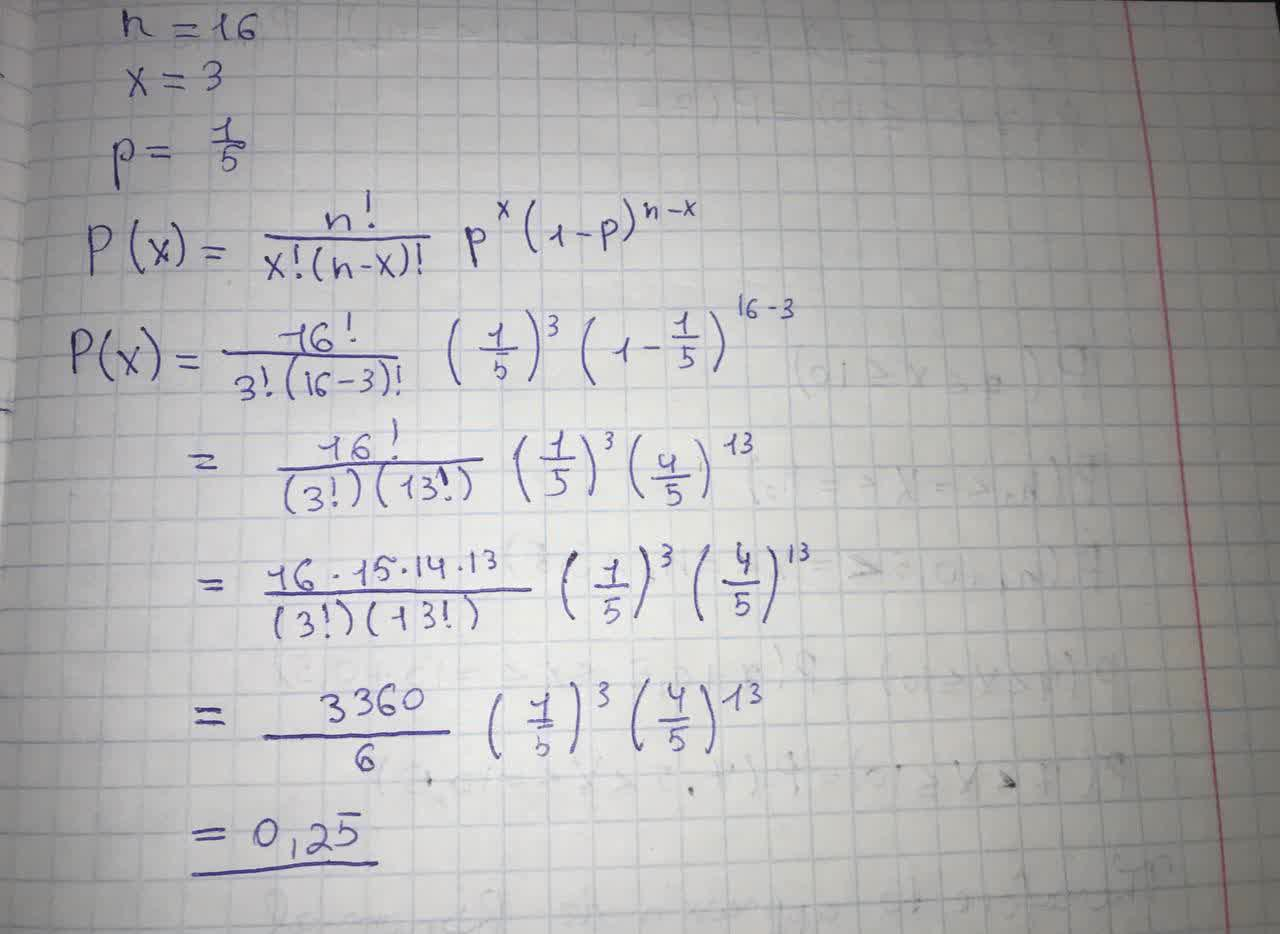# Use the binomial probability formula to find P(x) n= 16, x=3, p- 1/5Amari Flowers 2021-09-09 Answered

Use the binomial probability formula to find $P\left(x\right)$

You can still ask an expert for help

• Questions are typically answered in as fast as 30 minutes

Solve your problem for the price of one coffee

• Math expert for every subject
• Pay only if we can solve itdessinemoie